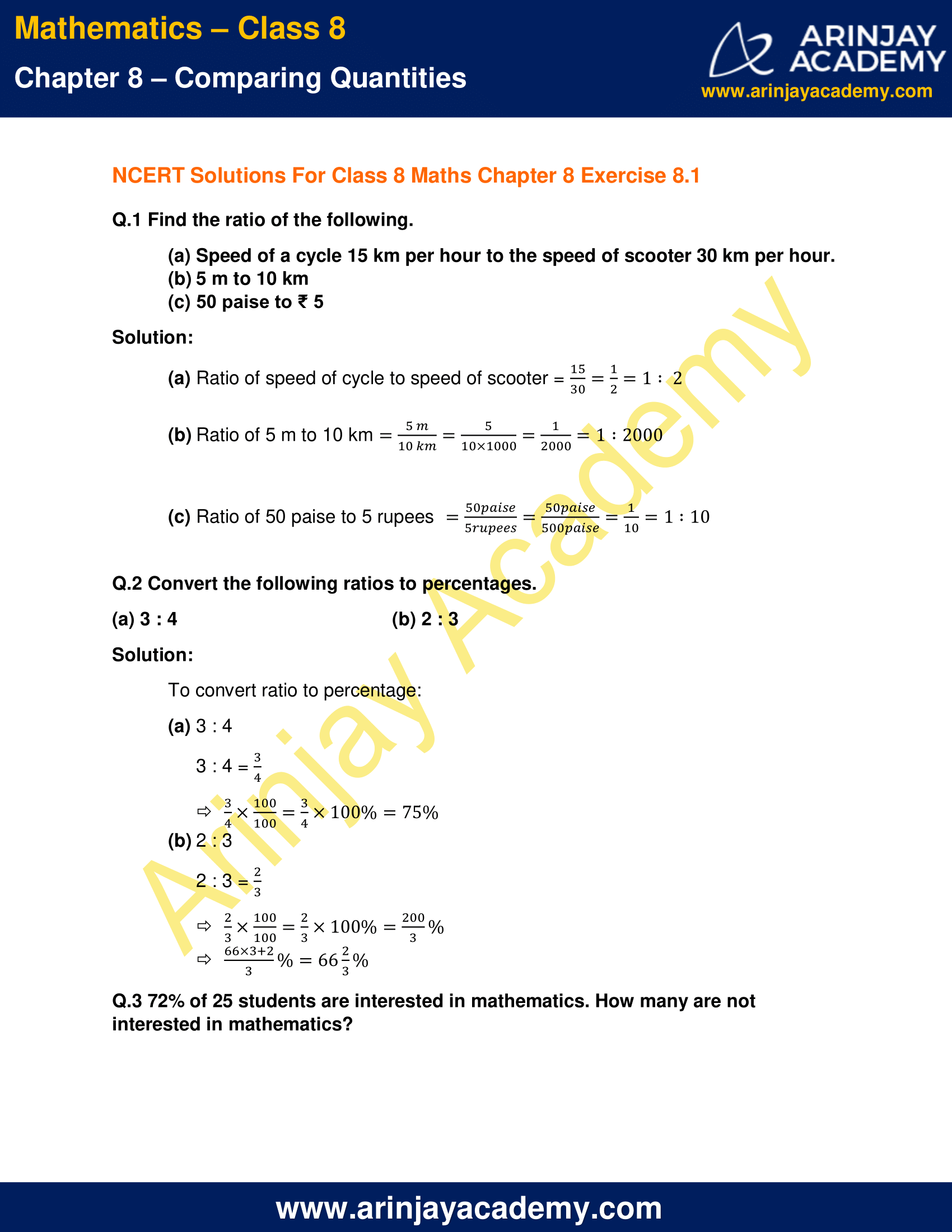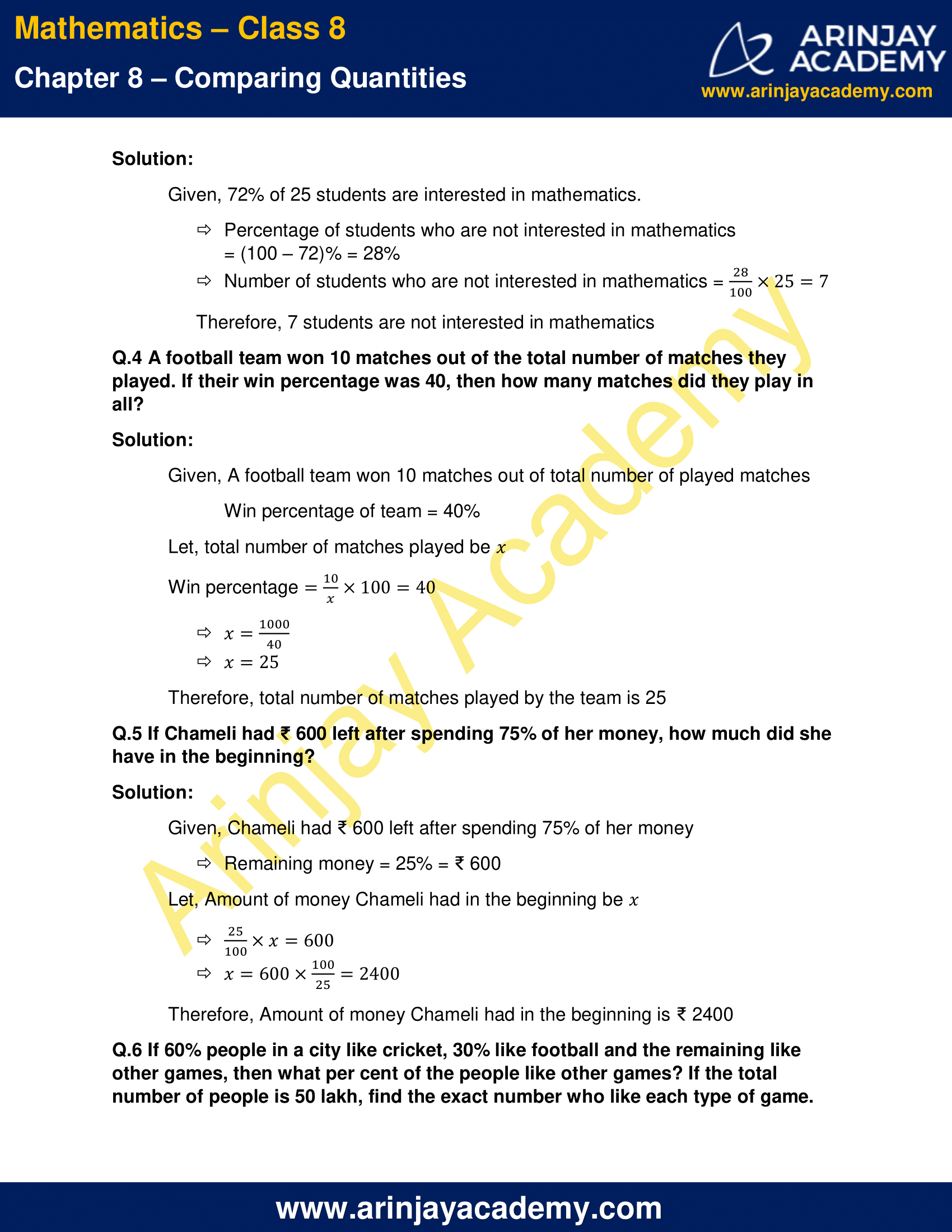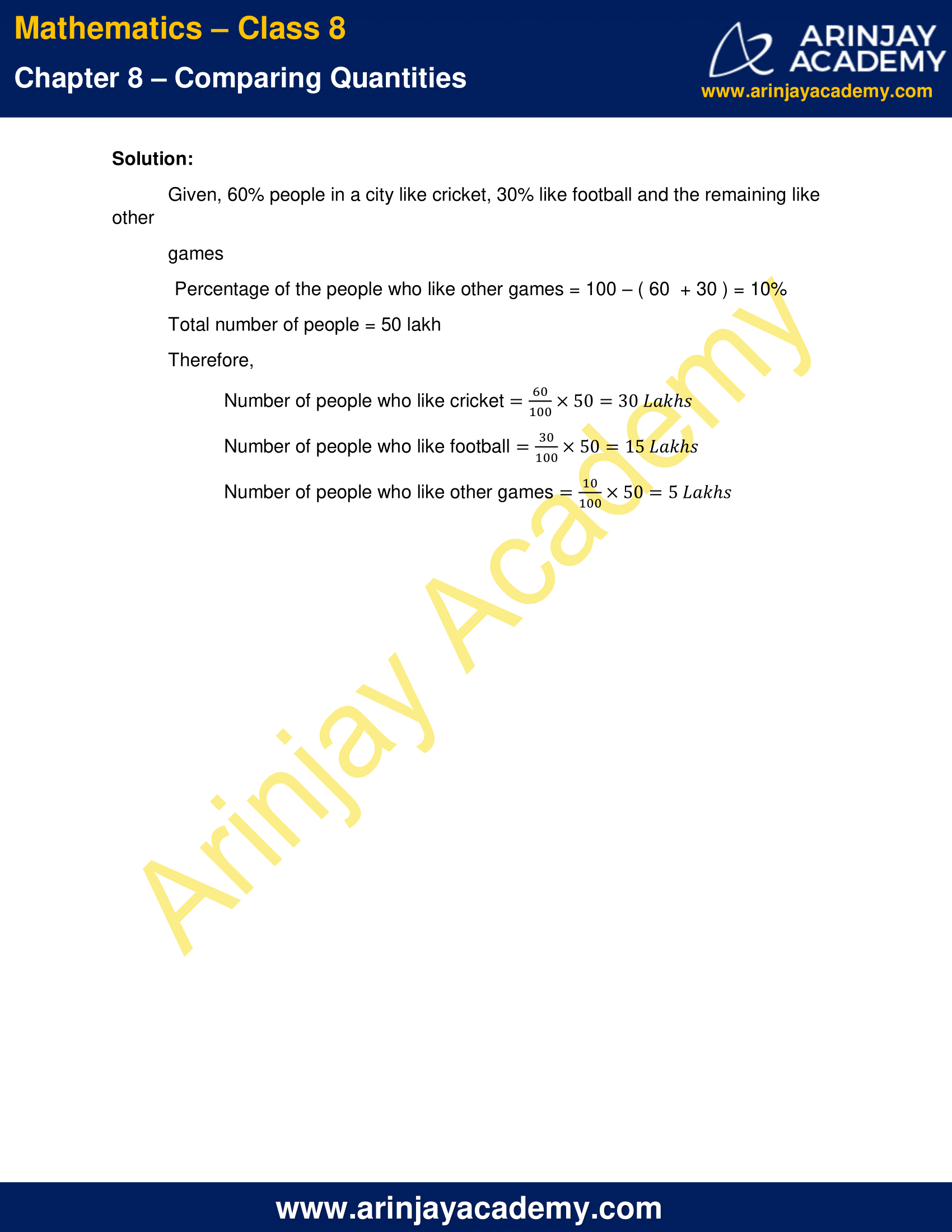# NCERT Solutions for Class 8 Maths Chapter 8 Exercise 8.1 – Comparing Quantities

NCERT Solutions for Class 8 Maths Chapter 8 Exercise 8.1 – Comparing Quantities, has been designed by the NCERT to test the knowledge of the student on the topic – Recalling Ratios and Percentages

### NCERT Solutions for Class 8 Maths Chapter 8 Exercise 8.1 – Comparing QuantitiesNCERT Solutions for Class 8 Maths Chapter 8 Exercise 8.1 – Comparing Quantities

Q.1 Find the ratio of the following.

(a) Speed of a cycle 15 km per hour to the speed of scooter 30 km per hour.
(b) 5 m to 10 km
(c) 50 paise to ₹ 5

Solution:

(a) Ratio of speed of cycle to speed of scooter = (15/30) = (1/2) = 1 : 2

(b) Ratio of 5 m to 10 km = (5m/10km) = (5/10 × 1000) = (1/2000) = 1 : 2000

(c) Ratio of 50 paise to 5 rupees = (50 paise/5 rupees) = (50 paise/500 paise) = (1 /10) = 1 : 10

Q.2 Convert the following ratios to percentages.

(a) 3 : 4
(b) 2 : 3

Solution:

To convert ratio to percentage:

(a) 3 : 4

3 : 4 = 3/4

(3/4) x (100/100)

= (3/4) x 100%

= 75%

(b) 2 : 3

2 : 3 = (2/3)

= (2/3) x (100/100)

= (2/3) x 100%

= (200/3) %

= [66 × (3+2)]/3 %

= 66 × (2/3) %

Q.3 72% of 25 students are interested in mathematics. How many are not interested in mathematics?

Solution:

Given, 72% of 25 students are interested in mathematics.

⇒Percentage of students who are not interested in mathematics

= (100 – 72)% = 28%

⇒Number of students who are not interested in mathematics = 28/100 x 25 = 7

Therefore, 7 students are not interested in mathematics

Q.4 A football team won 10 matches out of the total number of matches they played. If their win percentage was 40, then how many matches did they play in all?

Solution:

Given, A football team won 10 matches out of total number of played matches

Win percentage of team = 40%

Let, total number of matches played be x

Win percentage (10/x) × 100 = 40

x = (1000/40)

x = 25

Therefore, total number of matches played by the team is 25

Q.5 If Chameli had 600 left after spending 75% of her money, how much did she have in the beginning?

Solution:

Given, Chameli had ₹ 600 left after spending 75% of her money

Remaining money = 25% = ₹ 600

Let, Amount of money Chameli had in the beginning be

(25/100) × x = 600

x = 600 × (100/25) = 2400

Therefore, Amount of money Chameli had in the beginning is ₹ 2400

Q.6 If 60% people in a city like cricket, 30% like football and the remaining like other games, then what per cent of the people like other games? If the total number of people is 50 lakh, find the exact number who like each type of game.

Solution:

Given, 60% people in a city like cricket, 30% like football and the remaining like other games

Percentage of the people who like other games = 100 – ( 60  + 30 ) = 10%

Total number of people = 50 lakh

Therefore,

Number of people who like cricket = (60/100) x 50 = 30 Lakhs

Number of people who like football = (30/100) x 50 = 15 Lakhs

Number of people who like other games = (10/100) x 50 = 5 Lakhs

The next Exercise for NCERT Solutions for Class 8 Maths Chapter 8 Exercise 8.2 – Comparing Quantities can be accessed by clicking here

Download NCERT Solutions For Class 8 Maths Chapter 8 Exercise 8.1 – Comparing Quantities